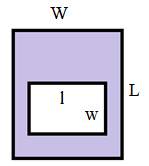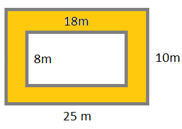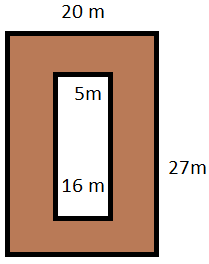# Area between two rectangles

#### Complete Python Prime Pack

9 Courses     2 eBooks

#### Artificial Intelligence & Machine Learning Prime Pack

6 Courses     1 eBooks

#### Java Prime Pack

9 Courses     2 eBooks

In this lesson, we solve problems involving areas between two rectangles.

A rectangle is given and inside it a smaller rectangle is drawn and then the area between these rectangles is found as follows. Consider the following figure. There is a rectangle L × W. Inside it there is another rectangle l × w. The area between these rectangles is shaded. We find the area of this shaded region.Formula for finding area between rectangles

Area between rectangles

= Area of bigger rectangle – Area of smaller rectangle

= L × W – l × w

Where the length and width of bigger rectangle are L and W and

Length and width of the smaller rectangle are l and w.

Find the area between the following pair of rectangles.### Solution

Step 1:

Area of a rectangle = l × w; l = length; w = width

Step 2:

Area between the given pair of rectangles = 25 × 10 − 18 × 8

= 250 – 144

= 106 square m

Find the area between the following pair of rectangles.### Solution

Step 1:

Area of a rectangle = l × w; l = length; w = width

Step 2:

Area between the given pair of rectangles = 27 × 20 − 16 × 5

= 540 – 80

= 460 square m